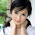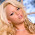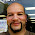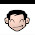Wednesday, November 16, 2011

HP 15C Programming Tutorial - Part 7: Solve and Integration

Solving Equations with the HP 15C

The HP 15C has a powerful solve feature that finds the real roots of the equation f(x)=0 given an interval.

Caution: When using the solve feature on an original HP 15C calculator - you will want to allow additional time since the calculator can be slow in the solve process.

Procedure:
1. Program an equation and label it.
2. In Run mode, enter the lower limit of the interval and press [ENTER].
3. Enter the upper limit of the interval, and press [ f ] [ ÷ ] (SOLVE) Label. Label can be 0-9, .0-.9, or A-E.

We will use two examples to illustrate the power of the SOLVE function.

Example 1

Find the root for the equation f(x) = x ln x - 3. We claim that the root is somewhere in the interval [1, 6].

First enter the equation. For this equation, use Label 0. Assume that x is in the display.

Key Codes Key
001 42 21 0 LBL 0
002 36 ENTER * duplicates x
003 43 12 LN * X: ln(x), Y: x
004 20 × * x ln (x)
005 3 3
006 30 - * x ln (x) - 3
007 43 32 RTN

1. Press [ g ] [R/S] (P/R) to go to Run Mode.
2. Press [ 1 ] [ENTER] [ 6 ] [ f ] [ ÷ ] (SOLVE) [ 0 ].

Result: x ≈ 2.8574.

Example 2

Find the two roots to the equation g(x) = x^2 + 4x - 3. Using Horner's Method, we can rewrite the equation as g(x) = (x + 4)x - 3.

We will use Label 1 for g(x).

Key Codes Key
001 42 21 1 LBL 1
002 36 ENTER * duplicates x
003 4 4
004 40 +
005 20 × * (x + 4)x
006 3 3
007 30 - * (x + 4)x - 3
008 43 32 RTN

Let's try to see if we can find a positive root. How about an interval of [0, 3]?

0 [ENTER] 3 [ f ] [ ÷ ] (SOLVE) 1

Result: x ≈ 0.6458.

Are there any roots bigger than 0.6458? Try an interval of [3, 100].

3 [ENTER] 100 [ f ] [ ÷ ] (SOLVE) 1

Result: x ≈ 0.6458 (no different)

How about any roots below 0? Try an interval [-100, 0].

100 [CHS] [ENTER] 0 [ f ] [ ÷ ] (SOLVE) 1

Result: x ≈ -4.6458

So our two roots are x ≈ {-4.6458, 0.6458}.

Integral

The HP 15C also has a built in integral function ( ∫ ). This is useful for finding definite integrals for functions, even when the function is not defined at the end points.

Caution: Just like the Solve function - allow extra time with the Integral function when using the original HP 15C calculators. In addition, using the Integral function requires 23 memory registers to operate. It may be a good idea to clear the program memory prior to entering functions for integration.

Procedure:
1. Enter a function in a program. The program must have a label.
2. Enter the lower limit of integration and press [ENTER].
3. Enter the upper limit of integration and press [ f ] [ × ] ( ∫ ).

We will use two examples to illustrate the Integration feature.

Example 1

Calculate ∫ ( e ^ (-x^2), x, 0, 2). Use Label 2.

Key Code Keys
001 42 21 2 LBL 2
002 43 11 x^2
003 16 CHS
004 12 e^x * e^(-x^2)
005 43 32 RTN

Press [ g ] [R/S] (P/R) to switch to Run Mode. Then press [ 0 ] [ENTER] [ 2 ] [ f ] [ × ] ( ∫ ) [ 2 ].

Result: Integral ≈ 0.8821

Example 2

Calculate ∫ ( |sin x|, x, 0, 2π). Use Label 3.

Key Code Keys
001 42 21 3 LBL 3
002 43 8 RAD * use Radians mode
003 23 SIN
004 43 16 ABS * |sin x|
005 43 32 RTN

In Run mode:

0 [ENTER] [ g ] [EEX] ( π ) 2 [ × ] [ f ] [ × ] ( ∫ ) 3

Result: Integral = 4

This concludes Part 7 of the tutorial. In the next part we will use the knowledge of programming functions and equations to calculate derivatives and sums.

This tutorial is property of Edward Shore. © 2011

1.Hi... that was great stuff.. I really like reading on this subject Could you tell me more on that... I love to explore

PPI Refund

2.Your blogs are totally worth gift quantify and liveliness.
ppi calculator

1.Thank you Frencis. Much appreciated.

Eddie

3.This is an informative blog by which I have got that info which I really wanted to get. free ppi calculator

4.I really enjoy reading and also appreciate your work. read more here - ppiclaimscalculator.org.uk

5.Is there any further reading you would recommend on this?

Amela
can I get a ppi refund

1.If it is available, the HP 15C Advanced Functions Handbook (now a pdf file). You can also check the MoHPC forums (http://www.hpmuseum.org/forum/index.php) for additional information regarding the HP 15C as it is sometimes discussed.

Hope this helps,

Eddie

2.If it is available, the HP 15C Advanced Functions Handbook (now a pdf file). You can also check the MoHPC forums (http://www.hpmuseum.org/forum/index.php) for additional information regarding the HP 15C as it is sometimes discussed.

Hope this helps,

Eddie

6.Matrix and Solve features require a lot of Memory Registers, in order to do those calculation you must clear all Memory which is a draw back if you already have some important program that already in it. The App version should put external program memory slot for later use so that you don't have to use up the memory register.

7.I only got the HP-15C for Andriod on Galaxy S7 Edge the screen size is perfect almost the same dimension as the real one. With this App all calculation give result very fast. Even try to do the Golden Ratio to convert the decimal to fraction in full decimal digits 1.618033989 to fraction 75025/46368 or pi = 104348/33215

8.Great stuff, thanks! Best to do with your HP 15C (app) in your hands, so that you can try it out directly :-)

9.X^2+4X-3=0
can be program as [X^2][LSTx] 4 x 3 -

1.you neglected the addition which should follow the multiply!

HP 12C: Finding Two Factors of an Integer

HP 12C:  Finding Two Factors of an Integer  Introduction This program finds two factors of the integer N, where one of the factors ...